Mental Maths TestPage 1

WATCH ALL SLIDES

Slide 1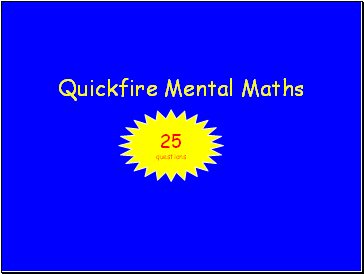Quickfire Mental Maths

25

questions

Slide 2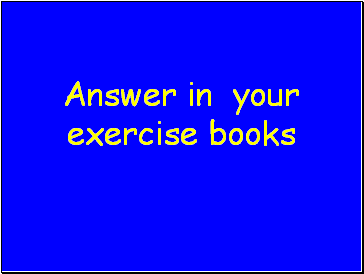Slide 3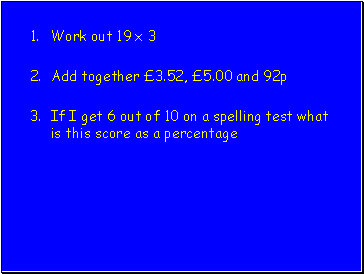Work out 19 x 3

Add together £3.52, £5.00 and 92p

If I get 6 out of 10 on a spelling test what is this score as a percentage

Slide 4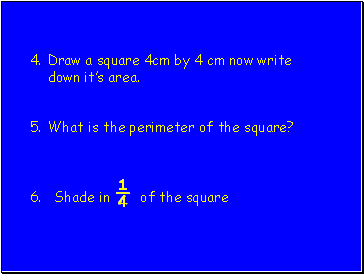Draw a square 4cm by 4 cm now write down it’s area.

What is the perimeter of the square?

6. Shade in ¼ of the square

Slide 5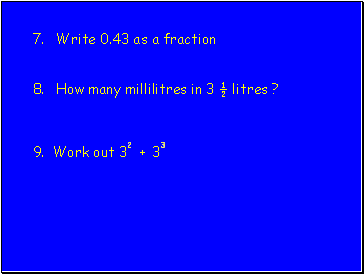Write 0.43 as a fraction

How many millilitres in 3 ½ litres ?

9. Work out 32 + 33

Slide 6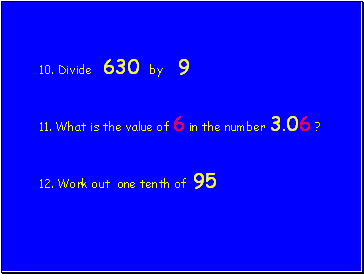10. Divide 630 by 9

11. What is the value of 6 in the number 3.06 ?

12. Work out one tenth of 95

Slide 7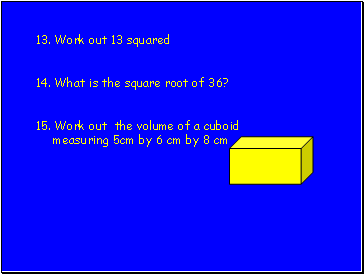13. Work out 13 squared

14. What is the square root of 36?

15. Work out the volume of a cuboid measuring 5cm by 6 cm by 8 cm

Slide 8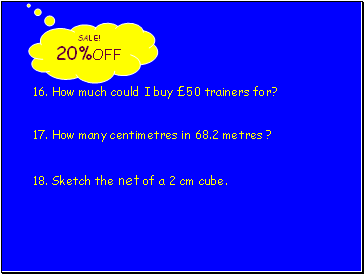16. How much could I buy £50 trainers for?

17. How many centimetres in 68.2 metres ?

18. Sketch the net of a 2 cm cube.

SALE!

20%OFF

Slide 9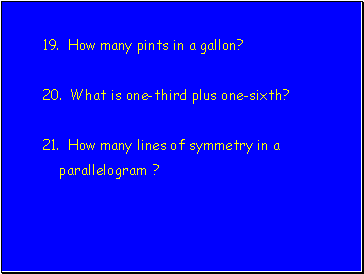19. How many pints in a gallon?

20. What is one-third plus one-sixth?

21. How many lines of symmetry in a parallelogram ?

Slide 10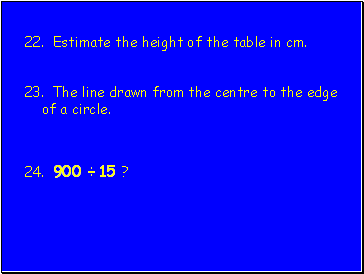22. Estimate the height of the table in cm.

23. The line drawn from the centre to the edge of a circle.

24. 900 ÷ 15 ?

Slide 11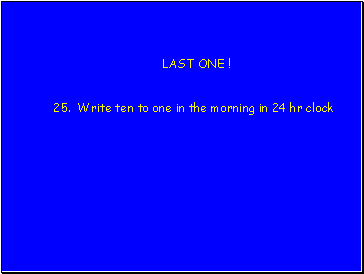LAST ONE !

25. Write ten to one in the morning in 24 hr clock

Slide 12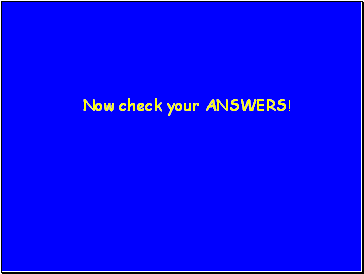Slide 13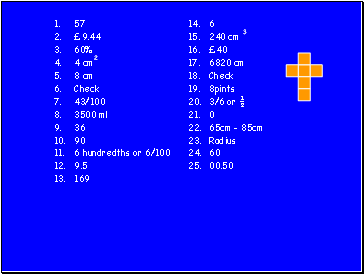57

£9.44

60%

4 cm2

8 cm

Check

43/100

3500 ml

36

90

6 hundredths or 6/100

9.5

169

6

240 cm 3

£40

6820 cm

Check

8pints

3/6 or ½

0

65cm – 85cm

60

00.50

Slide 14This powerpoint was kindly donated to www.worldofteaching.com

Go to page:
1  2